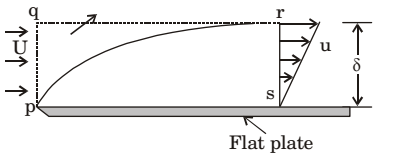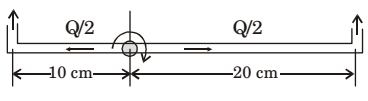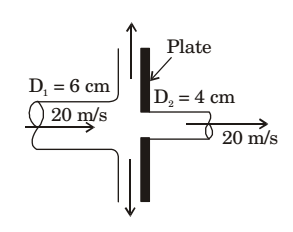## Fluid Mechanics and Hydraulic Machinery Miscellaneous

#### Fluid Mechanics and Hydraulic Machinery

Direction: A smooth flat plate with a sharp leading edge is placed along a gas stream flowing at U = 10 m/s. The thickness of the boundary layer at section r – s is 10 mm, the breadth of the plate is 1 m (into the paper) and the density of the gas, ρ = 1.0 kg/m3. Assume that the boundary layer is thin, two-dimensional, and follows a linear velocity distribution, u = U (y/δ), at the section r–s, where y is the height from plate.1. The integrated drag force (in N) on the plate, between p – s, is

1. By momentum equation, we can find drag force.

##### Correct Option: C

By momentum equation, we can find drag force.

1. The mass flow rate (in kg/s) across the section q – r is

1. Mass entering from side q-p = Mass leaving from side q-r + Mass leaving the side r-s

##### Correct Option: B

Mass entering from side q-p = Mass leaving from side q-r + Mass leaving the side r-s

1. A sprinkler shown in the figure rotates about its hinge point in a horizontal plane due to water flow discharged through its two exit nozzles.The total flow rate Q through the sprinkler is 1 litre/sec and the cross-sectional area of each exit nozzle is 1 cm². Assuming equal flow rate through both arms and a frictionless hinge, the steady state angular speed of rotation (rad/s) of the sprinkler is _______ (correct to two decimal places).

1. NA

##### Correct Option: A

NA

1. A 60 mm diameter water jet strikes a plate containing a hole of 40 mm diameter as shown in the figure. Part of the jet passes through the hole horizontally, and the remaining is deflected vertically. The density of water is 1000 kg/m3. If velocities are as indicated in the figure, the magnitude of horizontal force (in N) required to hold the plate is _________.1. NA

##### Correct Option: A

NA

1. An ideal water jet with volume flow rate of 0.05 m3/s strikes a flat plate placed normal to its path and exerts a force of 1000 N. Considering the density of water as 1000 kg/m3, the diameter (in mm) of the water jet is ______.

1. NA

NA